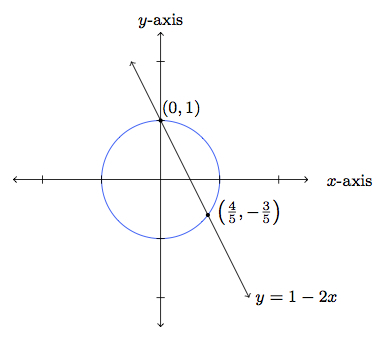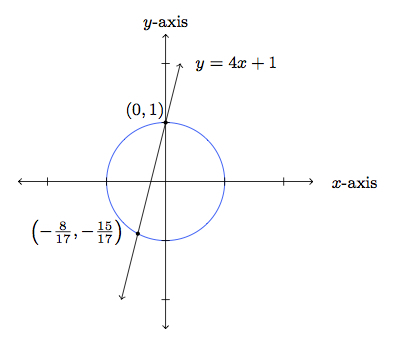# Pythagorean Triples

Alignments to Content Standards: A-REI.C.7

This task will investigate the intersection points of the circle $C$ of radius 1 centered at $(0,0)$ and different lines passing through the point $(0,1)$. Recall that $C$ is the set of solutions to the equation $x^2 + y^2 = 1$. Also recall that a Pythagorean Triple is a set of three positive whole numbers $a, b, c$ so that $a^2 + b^2 = c^2$.

1. Find the two points of intersection of the line $y = -2x+1$ and the circle of radius 1 with center $(0,0)$.
2. Find the two points of intersection of the line $y = 4x+1$ and the circle of radius 1 with center $(0,0)$.
3. Suppose $m$ is a (non-zero) rational number and we intersect the line defined by $y = mx + 1$ with the circle $C$. Explain why $(0,1)$ is one of the solutions and the other solution has rational numbers for both coordinates.
4. Parts (a), (b), and (c) produce a point on $C$ different from $(1,0)$. Explain how this point can be used to produce a Pythagorean triple.

## IM Commentary

This task has two separate goals: the first is to solve a pair of equations, one quadratic and the other linear. The context is geometric and the teacher may wish to do an example, like part (a) or (b), to help students visualize the situation. The second goal is to observe that a point, such as $\left( \frac{3}{5}, \frac{4}{5}\right)$, that lies on $C$ gives rise to a Pythagorean triple, in this case the triple $(3,4,5)$. It will be a challenge for students to solve the equations, visualize the points of intersection, and understand the connection with Pythagorean triples. Therefore they are encouraged to work on this task in groups and the teacher may wish to provide extra guidance. In addition to working through an example like parts (a) and (b), the teacher may wish to have the students think about other Pythagorean triples, such as $(5,12,13)$, and find the equation of the line that contains this point and $(1,0)$. This shows that the process works in both directions: a line with rational slope containing $(1,0)$ gives a Pythagorean triple, and a Pythagorean triple gives a line with rational slope passing through $(1,0)$.

For part (d), the teacher may wish to give the students some explicit points on $C$, such as $\left(\frac{5}{13},\frac{12}{13}\right)$ or $\left(\frac{8}{17}, \frac{15}{17}\right)$ to help them see in an explicit case how to get a Pythagorean triple, (5,12,13) in the first case and (8,15,17) in the second case. This will provide them with confidence manipulating the algebraic expressions which they will need to do in part (d).

The reason why the equations for the lines in parts (a) and (b) are not simpler is that the lines with slope $\pm 1$ lead to solutions with $y$ coordinate 0 and the lines with slope $\pm 2, \pm \frac{1}{2}$ and $\pm 3, \pm \frac{1}{3}$ all lead to the same Pythagorean triple (3,4,5), with different signs on the 3 and 4. If class time is available, teachers may wish to have students experiment, ideally using technology, with different lines. In addition to the ''standard'' Pythagorean triple (3,4,5), this will produce many with which students are not familiar. If students are very comfortable and confident with the mathematics of this task, they could have a competition to see who can create the most Pythagorean triples or the largest Pythagorean triple.

This task should be compared with www.illustrativemathematics.org/illustrations/594 which has a similar goal but achieves that purpose through algebraic reasoning as opposed to the geometric point of view adopted here. This task can be carried further to show that all Pythagorean triples can be captured in this way by choosing different lines through the point $(0,1)$.

This task provides good opportunities for students to engage in MP2, Reason Abstractly and Quantitatively: this task requires solving a system of equations while also understanding why the solution is related to Pythagorean triples. Since the task is ideally suited for group work, it also provides a good opportunity for students to engage in MP3, Construct Viable Arguments and Critique the Reasoning of Others. It will likely require several students and different perspectives in order to fully grasp the mathematics behind this task.

## Solutions

Solution: 1 Using algebra to solve equations

1. The points of intersection of the line defined by $y = - 2x+1$ and $C$, defined by $x^2 + y^2 = 1$, can be found by substituting $y = - 2x+1$ into $x^2 + y^2 = 1$. This gives $$x^2 + (-2x+1)^2 = 1.$$ Expanding the right hand side and gathering like terms this is the same as $$5x^2 -4x + 1 = 1.$$ This means that $5x^2 - 4x = 0$ or $x(5x - 4) = 0$. This is true when $x = 0$ or when $x = \frac{4}{5}$. Plugging these $x$-values into the equation $y = - 2x+1$ we find that the two points of intersection of the line and circle are $(0,1)$ and $\left(\frac{4}{5},-\frac{3}{5}\right)$.

This is pictured below:Note that we know that the point (0,1) lies on the $C$ and on the line defined by $y = - 2x+1$ so the main purpose of the calculations is to find the other point of intersection.

2. The points of intersection of the line defined by $y = 4x+1$ and $C$, defined by $x^2 + y^2 = 1$ can be found by substituting $y = 4x+1$ into $x^2 + y^2 = 1$. This gives $$x^2 + (4x+1)^2 = 1.$$ Expanding the right hand side and gathering like terms this is the same as $$17x^2 +8x + 1 = 1.$$ This means that $17x^2 + 8x = 0$ or $x(17x + 8) = 0$. This is true when $x = 0$ or when $x = -\frac{8}{17}$. Plugging these $x$-values into the equation $y = 4x+1$ we find that the two points of intersection of the line and circle are $(0,1)$ and $\left(-\frac{8}{17},-\frac{15}{17}\right)$.

This is pictured below:As in part (a), it is $\left(-\frac{8}{17},-\frac{15}{17}\right)$ that is really the unknown point of intersection.

3. Since $m \neq 0$, the point $(0,1)$ is a solution to both $x^2 + y^2 = 1$ and $y = mx$ so it will always be a point of intersection of the graphs of these two equations. When we substitute $y = mx+1$ into $x^2 + y^2 = 1$ we find $$x^2 + (mx+1)^2 = 1$$ which reduces to $$(m^2 + 1)x^2 + 2mx + 1 = 1.$$ Subtracting 1 from both sides gives $$(m^2 + 1) x^2 + 2mx = 0.$$ This factors as $$x\left((m^2 + 1)x + 2m\right) = 0.$$ Solving gives us $x = 0$ and $x = \frac{-2m}{m^2+1}$. So the two points of intersection are $(0,1)$, which we already knew, and $\left(\frac{-2m}{m^2+1}, 1 - \frac{2m^2}{m^2+1}\right)$. Since $m$ is a non-zero rational number, this second point has rational numbers for both $x$ and $y$ coordinates and is a point different from (0,1) and from (1,0).

We can (and should) check that the general expression here gives the same answers that we found in parts (a) and (b) when we plug in $m= -2$ and $m = 4$ respectively. Below is a blown up picture of part (a), showing the right triangle with sides of lengths $\frac{3}{5}$, $\frac{4}{5}$, and $\frac{5}{5}$ to which the Pythagorean Theorem applies:After scaling by a factor of 5 this gives the Pythagorean triple (3,4,5).

4. Part (c) produces the pair of rational numbers $$\left(\frac{-2m}{m^2+1}, 1 - \frac{2m^2}{m^2+1}\right)$$ which lie on the circle defined by $x^2 + y^2 = 1$. We can find the explicit Pythagorean triple, in terms of $m$, by doing a little polynomial arithmetic. We can rewrite this point as $$\left(\frac{-2m}{m^2+1}, \frac{1-m^2}{m^2+1}\right)$$ We have $$\left(\frac{-2m}{m^2+1}\right)^2 + \left( \frac{1-m^2}{m^2+1}\right)^2 = 1$$ which gives, clearing the denominators on the left hand side, $$(-2m)^2 + (1-m^2)^2 = \left(m^2+1\right)^2.$$ For each integer value of $m$, this is a Pythagorean triple. If $m$ is rational number, there are more denominators to clear before this becomes a Pythagorean triple.

Solution: 2 Alternate solution to part (c): seeing structure in expressions

The quadratic formula tells us that the roots to the equation $ax^2 + bx + c = 0$ are $$x = \frac{-b \pm\sqrt{b^2 - 4ac}}{2a}.$$ If the coefficients $a,b,c$ are rational numbers then the only part of this expression that can fail to be a rational number is $\sqrt{b^2 -4ac}$. In our case, when we plug in $y = mx + 1$ to $x^2 + y^2 = 1$ this will be a quadratic polynomial in $x$ with rational coefficients. Since one of the solutions is $x = 0$ this means that $\sqrt{b^2 - 4ac}$ must be a rational number for this quadratic polynomial (if it were irrational then the entire expression for $x$ would be irrational). So the other root of this equation must also be a rational number. When we plug our rational solutions for $x$ into $y = mx + 1$ we get a rational value for $y$ and therefore the intersection points of the line and circle have rational coordinates.

This line of reasoning shows that the intersection points of the line and circle have rational coordinates without calculating those coordinates.